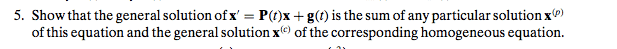# Sum of particular solution and homogenous solution of differential equation

## Homework Statement## The Attempt at a Solution

Suppose there is a solution $X^S ≠ X^p + X^c$. Let $Y_1,...,Y_n$ be the set of linearly independent solutions whose span gives the general solution to the differential equation. Let us write the general solution to the differential equation as a linear combination of the elements in this set: $c_1 Y_1 + ... c_n Y_n = X^G$. Then, for some choice of constants $a_1,...,a_n$, we have $X^S = a_1 Y_1 + ... + a_n Y_n$. Since an equation is either homogenous or nonhomogenous, these elements of the solution set must combine to give either the homogenous solution (when the equation is homogenous) or the particular solution (otherwise). Let the subset $Y_1,...,Y_j$ be the solutions that span the homogenous solutions and $Y_{j+1},...,Y_n$ be the solutions that span the particular solutions. Thus, we may write that, for some arbitrary constants $b_1,...,b_n$, we have $b_1 Y_1 + ... + b_j Y_j = X^c$ and for other constants $d_1,...,d_n$, we have $d_1 Y_1 + ... + d_j Y_j = X^p$. Writing these as a linear combination, we have, $X^c + X^p = (b_1 Y_1 + ... + b_j Y_j) + (d_1 Y_1 + ... + d_j Y_j)$, which, for the correct choices of constants, gives us the equation for $X^s$, which is contrary to our assumption that $X^s$ could not be written as the sum of the homogenous and particular solutions. This shows that all solutions can be given by the sum of the particular and homogenous solutions, and thus they give rise to the general solution.

Is this conceivably right?

Dick
Science Advisor
Homework Helper

## Homework Statement## The Attempt at a Solution

Suppose there is a solution $X^S ≠ X^p + X^c$. Let $Y_1,...,Y_n$ be the set of linearly independent solutions whose span gives the general solution to the differential equation. Let us write the general solution to the differential equation as a linear combination of the elements in this set: $c_1 Y_1 + ... c_n Y_n = X^G$. Then, for some choice of constants $a_1,...,a_n$, we have $X^S = a_1 Y_1 + ... + a_n Y_n$. Since an equation is either homogenous or nonhomogenous, these elements of the solution set must combine to give either the homogenous solution (when the equation is homogenous) or the particular solution (otherwise). Let the subset $Y_1,...,Y_j$ be the solutions that span the homogenous solutions and $Y_{j+1},...,Y_n$ be the solutions that span the particular solutions. Thus, we may write that, for some arbitrary constants $b_1,...,b_n$, we have $b_1 Y_1 + ... + b_j Y_j = X^c$ and for other constants $d_1,...,d_n$, we have $d_1 Y_1 + ... + d_j Y_j = X^p$. Writing these as a linear combination, we have, $X^c + X^p = (b_1 Y_1 + ... + b_j Y_j) + (d_1 Y_1 + ... + d_j Y_j)$, which, for the correct choices of constants, gives us the equation for $X^s$, which is contrary to our assumption that $X^s$ could not be written as the sum of the homogenous and particular solutions. This shows that all solutions can be given by the sum of the particular and homogenous solutions, and thus they give rise to the general solution.

Is this conceivably right?

If you have to ask it's probably not right. That's just a bunch of general gibberish. You have to be specific. Start by saying exactly what is the homogeneous equation you are talking about.

LCKurtz
Science Advisor
Homework Helper
Gold Member
Look at my post #9 in this thread:

https://www.physicsforums.com/showthread.php?t=598602

It talks about this issue for a second degree scalar equation, but you might be able to adapt the ideas to your problem.